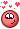## Recommended Posts

Posted (edited)

Best approach to make something rolling on Spline with provided vex code and file inside some Structure..Thanx ...FOund some pictures and codes Asia Forums ...HOW??

```
float theta = atan2(v@N.y, sqrt(v@N.x * v@N.x + v@N.z * v@N.z));

float gravity = (9.8 / float(60 * 60)) * -1;

f@speed += gravity * sin(theta);

float friction = 1;
f@speed *= friction;

f@curvePos += f@speed;

float curveLength = primintrinsic(1, "measuredperimeter", 0);

float ratio = (f@curvePos / curveLength) % 1;
vector2 posuv = set(ratio, 0);

@P = primuv(1, "P", 0, posuv);
@N = primuv(1, "N", 0, posuv);
@up = primuv(1, "up", 0, posuv);```

Edited by Librarian
•3

## Create an account

Register a new account